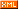SUMTEC -- There's a thing in my bloglet.

But it's not only one. It's many. It's the same as other things but it exactly likes nothing else...

 博客园 :: 首页 :: 博问 :: 闪存 :: 新随笔 :: 联系 :: 订阅:: 管理 ::http://blog.joycode.com/zhanbos/archive/2004/10/26/36605.aspxpublic void Test(){{int a;}{int a;}}.method public hidebysig instance void Test() cil managed{// Code Size: 2 byte(s).maxstack 0.locals (int32 num1,int32 num2)L_0000: nopL_0001: ret}public int Test(){int b;b
= new Random().Next(5);if (b < 5){int a = new Random().Next(5);Console.WriteLine(a);b
= a;}else{int a = new Random().Next(10);Console.WriteLine(a);b
= a;}return b;}Reflector 反编译结果：public int Test(){int num1 = new Random().Next(5);if (num1 < 5){int num2 = new Random().Next(5);Console.WriteLine(num2);return num2;}int num3 = new Random().Next(10);Console.WriteLine(num3);return num3;}public void Test(){{int a;}{int a;}int a;}

error CS0136: A local variable named 'a' cannot be declared in this scope because it would give a different meaning to 'a', which is already used in a 'child' scope to denote something elsepublic void Test(){a
= 2;{int a;}{int a;}int a;}

error CS0103: The name 'a' does not exist in the class or namespace 'ConsoleApplication1.Class2'

posted on 2004-10-26 13:05  Sumtec  阅读(4216)  评论(12编辑  收藏  举报